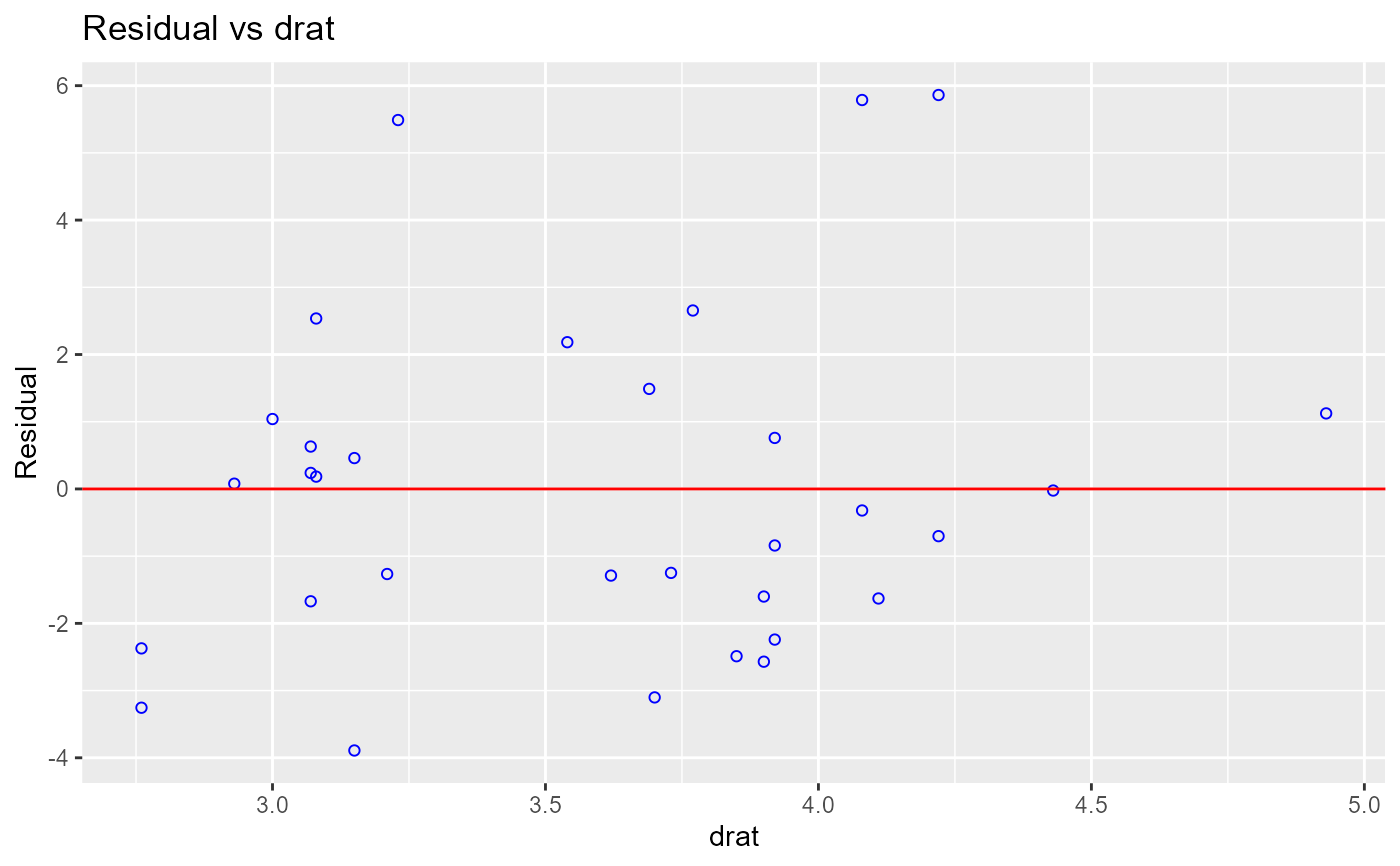Graph to determine whether we should add a new predictor to the model already containing other predictors. The residuals from the model is regressed on the new predictor and if the plot shows non random pattern, you should consider adding the new predictor to the model.

## Usage

rvsr_plot_shiny(model, data, variable, print_plot = TRUE)

## Arguments

model

An object of class lm.

data

A data.frame or tibble.

variable

Character; new predictor to be added to the model.

print_plot

logical; if TRUE, prints the plot else returns a plot object.

## Examples

model <- lm(mpg ~ disp + hp + wt, data = mtcars)
rvsr_plot_shiny(model, mtcars, 'drat')